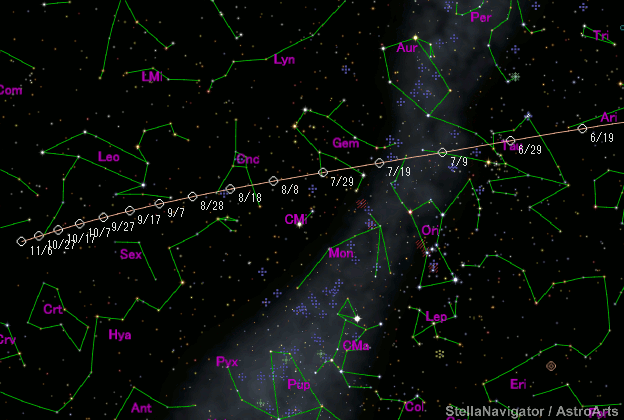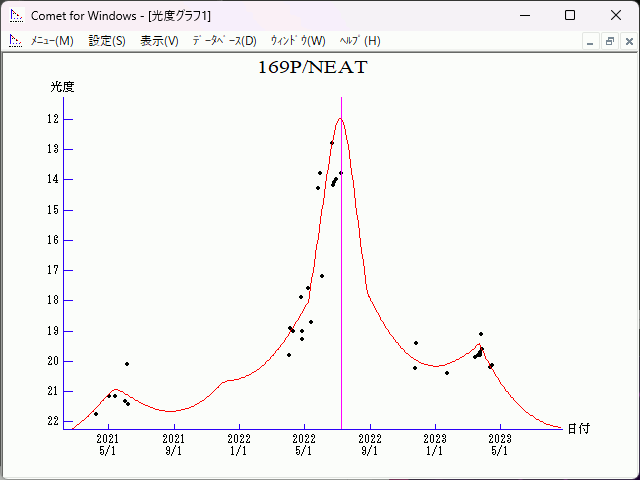# \$B%K!<%HWB@1(B

169P/NEAT (2022)###\$B50F;MWAG(B

```Epoch 2022 June 30.0 TT = JDT 2459760.5
T 2022 July  9.68361 TT                                 Rudenko
q   0.6028269            (2000.0)            P               Q
n   0.23478522     Peri.  218.04392     +0.82675419     -0.56240602
a   2.6022892      Node   176.10665     +0.55214239     +0.80668183
e   0.7683475      Incl.   11.29902     +0.10777889     +0.18155962
P   4.20
From 1186 observations 1989 Mar. 7-2020 May 13, mean residual 0".5.
```

###\$B@1?^(B###\$B8wEYJQ2=(B

```        H = 15.3  G = 0.15                [   ,-61]  (              \$B!A(B2022\$BG/(B 5\$B7n(B 9\$BF|(B)
m1 = 15.8 + 5 log\$B&\$(B + 20.0 log r  [-61, 50]  (2022\$BG/(B 5\$B7n(B 9\$BF|!A(B2022\$BG/(B 8\$B7n(B28\$BF|(B)
H = 15.3  G = 0.15                [ 50,   ]  (2022\$BG/(B 8\$B7n(B28\$BF|!A(B              )
```##### \$B50F;MWAG\$O(BM.P.E.C. 2020-K117\$B\$K7G:\\$5\$l\$?\$b\$N\$G\$9!#(B \$B@1?^\$O%9%F%i%J%S%2!<%?(B Ver.10 (\$B%"%9%H%m%"!<%D(B) \$B\$G:n@.\$7\$?\$b\$N\$G\$9!#(B \$B8wEY%0%i%U\$O(BComet for Windows\$B\$G:n@.\$7\$?\$b\$N\$G\$9!#(B